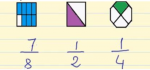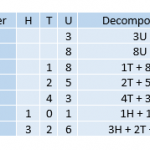Smartick is an advanced online program that teaches kids math and coding in only 15 min. a day

Jul18

## USA Team Wins Math OLI for the Second Consecutive YearAmerican high school mathletes have done it again! This is the second year in a row that the USA Team claimed the championship title at the International Mathematical Olympiad. The most prestigious problem-solving competition in the world, the 57th IMO was held July 6-16, 2016 in Hong Kong. Last summer was the first time since […]

Jul15

## Understand What a Fraction Is and When It Is UsedWith this post, we are going to understand the notion of a fraction as a part of a whole, as a division of natural numbers and its position on the number line. What is a fraction? A fraction represents the number of parts that we have of a whole that is divided into equal parts. Fractions […]

Jul14

## Combined Operations ProblemsHave you tried to do our combined operations problems? When we do a problem, there are times when there is no need to do any operation and other times when you have to do one or many. Today we are going to see these problems where various operations are necessary in order to arrive at a […]

Jul13

## Direct Proportions: What Are They? What Are They Used For?Today we are going to learn what direct proportions are and what they are used for. Before we go on, we need to know what a magnitude is. A magnitude is an amount that can be measured or counted. For example, the weight of a person, the number of construction workers working, the number of bananas, the […]

Jul11

## Geometric Figures and Straight LinesStraight lines are key to recognizing shapes that we often see in the real world: geometric figures. In today’s post, we will classify geometric figures formed from straight lines and think about where we can find them. We’ll start with the simplest: Two Straight LinesCan you make a figure with two straight lines? Well, of […]

Jul08

## Practice Adding Fractions with Examples

Today, we’re going to walk through some examples of adding fractions. Before you read this post, you might want to check out a previous post where we explain how to add fractions step by step. Let’s begin with the simplest of examples: Adding Fractions with the Same Denominator For example: The only thing we need […]

Jul07

## Units, Tens, Hundreds. Learn How They Are UsedIn today’s post, we are going to review Units, Tens, Hundreds, and see some examples of how they are used. Units It is the smallest natural number. We are going to represent a unit with a cube: To abbreviate the word unit, we will write U, for example: Tens Let’s look at a larger number […]

Jul06

## How to Add with and without Regrouping

In this post, we are going to learn how to do addition problems with and without regrouping. How to Add without Regrouping Place the addends one on top of the other so that the place values fall in the same columns. Add each column together separately, starting with the 1s place. The sums go below each column, […]

Jul04

## How to Subtract with and without Borrowing

In this post we will learn to subtract with and without borrowing. Subtract Without Borrowing: The steps we need to follow are: Place the number being subtracted under the number being subtracted from so that place values in the same column match.  Subtract each column separately starting with the ones Write the result of the subtraction […]

Jun30

## Rule of 3: Direct and InverseIn today’s post, we are going to work on proportions. This time we will look at a way of solving direct and inverse proportions: the rule of 3. What is the rule of 3? The rule of 3 is an operation that helps us quickly solve both direct and inverse proportion word problems. In order […]